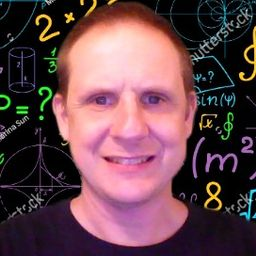# Donald A.

Lehigh University

## Biography

I am currently a secondary education math teacher. However, I've taught all grade levels including elementary, middle school, high school, and community college. I pray that you find my content helpful.

## Education

MS Mechanical Engineering
Lehigh University
MS Curriculum & Instruction
Shippensburg University of Pennsylvania
BS Engineering Science
Pennsylvania State University

## Topics Covered

Sampling and Data
Probability Topics
Probability and Counting Rules
Parametric Equations
Polar Coordinates
Trigonometry
Introduction to Vectors
Complex Numbers
Matrices
Rational Functions
Systems of Equations and Inequalities
Introduction to Matrices
Functions
Linear Functions
Polynomials
Work
Kinetic Energy
Potential Energy
Energy Conservation
Moment, Impulse, and Collisions
Applying Newton's Laws
Rotation of Rigid Bodies
Dynamics of Rotational Motion
Equilibrium and Elasticity
Gravitation
Thermal Properties of Matter
The First Law of Thermodynamics
The Second Law of Thermodynamics
Electromagnetic Waves
Wave Optics
Magnetic Field and Magnetic Forces
Mechanical Waves
Reflection and Refraction of Light
Temperature and Heat
Current, Resistance, and Electromotive Force
Direct-Current Circuits
Electromagnetic Induction
Alternating Current
Sampling and Simulation
Linear Regression and Correlation
Physics Basics
Motion Along a Straight Line
Motion in 2d or 3d
Sources of Magnetic field
Inductance
Derivatives
Differentiation
Applications of the Derivative
Newton's Laws of Motion
Fluid Mechanics
Electric Charge and Electric Field
Periodic Motion
Sound and Hearing
1 2 3 4 5 ... 500